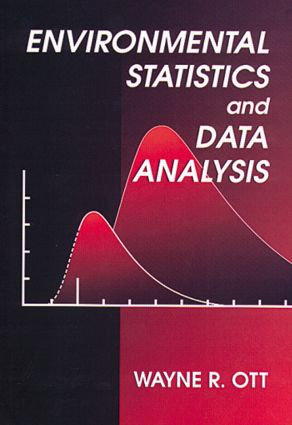Environmental Statistics and Data Analysis

1st Edition

CRC Press

336 pages

Purchasing Options:\$ = USD
Hardback: 9780873718486
pub: 1994-12-20
SAVE ~\$31.00
\$155.00
\$124.00
x
eBook (VitalSource) : 9780203756843
pub: 2018-12-13
from \$77.50

FREE Standard Shipping!

Description

This easy-to-understand introduction emphasizes the areas of probability theory and statistics that are important in environmental monitoring, data analysis, research, environmental field surveys, and environmental decision making. It communicates basic statistical theory with very little abstract mathematical notation, but without omitting important details and assumptions.

Topics include Bayes' Theorem, geometric distribution, computer simulation, histograms and frequency plots, maximum likelihood estimation, the tail exponential method, Bernoulli processes, Poisson processes, diffusion and dispersion of pollutants, normal distribution, confidence intervals, and stochastic dilution; gamma, chi-square, and Weibull distributions; and the two- and three-parameter lognormal distributions. The author also presents the Statistical Theory of Rollback, which allows data analysts and regulatory officials to estimate the effect of different emission control strategies on environmental quality frequency distributions.

Assuming only a basic knowledge of algebra and calculus, Environmental Statistics and Data Analysis provides an outstanding reference and collection of statistical procedures for analyzing environmental data and making accurate environmental predictions.

Reviews

"… provides a lucid explanation of how environmental processes can yield observations realized from various probability models, and hence gives better justification for their choice than empirical fit."

-Journal of the American Statistical Association

Random Processes

Stochastic Processes in the Environment

Structure of the Book

Theory of Probability

Probability Concepts

Probability Laws

Conditional Probability and Bayes' Theorem

Summary

Problems

Probability Models

Discrete Probability Models

Continuous Random Variables

Moments, Expected Value, and Central Tendency

Variance, Kurtosis, and Skewness

Analysis of Observed Data

Summary

Problems

Bernoulli Processes

Conditions for Bernoulli Process

Development of Model

Binomial Distribution

Applications to Environmental Problems

Computation of B(n,p)

Problems

Poisson Processes

Conditions for Poisson Process

Development of Model

Poisson Distribution

Examples

Applications to Environmental Problems

Computation of P(l,t)

Problems

Diffusion and Dispersion of Pollutants

Wedge Machine

Particle Frame Machine

Plume Model

Summary and Conclusions

Problems

Normal Processes

Conditions for Normal Process

Development of Model

Confidence Intervals

Applications to Environmental Problems

Computation of N(m,s)

Problems

Dilution of Pollutants

Deterministic Dilution

Stochastic Dilution

Applications to Environmental Problems

Summary and Conclusions

Problems

Lognormal Processes

Conditions for Lognormal Process

Development of Model

Lognormal Probability Model

Estimating Parameters of the Lognormal Distribution

Three-Parameter Lognormal Model

Statistical Theory of Rollback

Applications to Environmental Problems

Summary and Conclusions

Problems

Index